#### 推荐系统有哪些常用的评价标准

统计/机器学习 推荐系统 模型验证    浏览次数：15088        分享

20机器小白   2017-03-05 12:12

##### 4个回答
29

1. 精度Precision：P(k)

$$P(k) = \frac{c}{k}$$

2. 平均精度Average Precision: ap@n

$$ap@n=\sum_{k=1}^n \frac{P(k)}{\min(m,n)}$$

n是被预测的链接的总数，m是用户点击的链接的总数。

$ap@10=(1/1+2/4)/4\approx 0.38$

$ap@10=(1/2+2/3+3/5)/6\approx 0.29$

$ap@10=(1/2+2/7)/2\approx 0.39$

$ap@5=(1/1+2/2+3/4)/5\approx 0.55$

3. 平均精度均值Mean Average Precision: MAP@n

MAP计算的是N个用户的平均精度的均值。

$$MAP@n=\sum_{i=1}^N\frac{(ap@n)_i}{N}$$

$$MAP@10=\frac{0.38+0.17+0.26}{3}\approx 0.27$$

--------------------补充一下，4月30号--------------------

SofaSofa数据科学社区DS面试题库 DS面经高代兄   2017-03-12 10:45

18

$$CG_k=\sum_{i=1}^k \text{rel}_i.$$

$\text{rel}_i$表示第$k$个物品的相关性。假设我们共推荐$k$个电影，$rel_i$可以是用户对第$i$部电影的评分。

$M_1$,$M_2$,$M_3$,$M_4$,$M_5$，

5, 3, 2, 1, 2

CG没有考虑推荐的次序，在此基础之后我们引入对物品顺序的考虑，就有了DCG(discounted CG)，折扣累积增益。公式如下：

$$DCG_k=\sum_{i=1}^k \frac{2^{\text{rel}_i}-1}{\log_2(i+1)}.$$

$M_1$,$M_2$,$M_3$,$M_4$,$M_5$，

5, 3, 2, 1, 2

------------更新-------------

SofaSofa数据科学社区DS面试题库 DS面经abuu   2017-09-28 09:56

12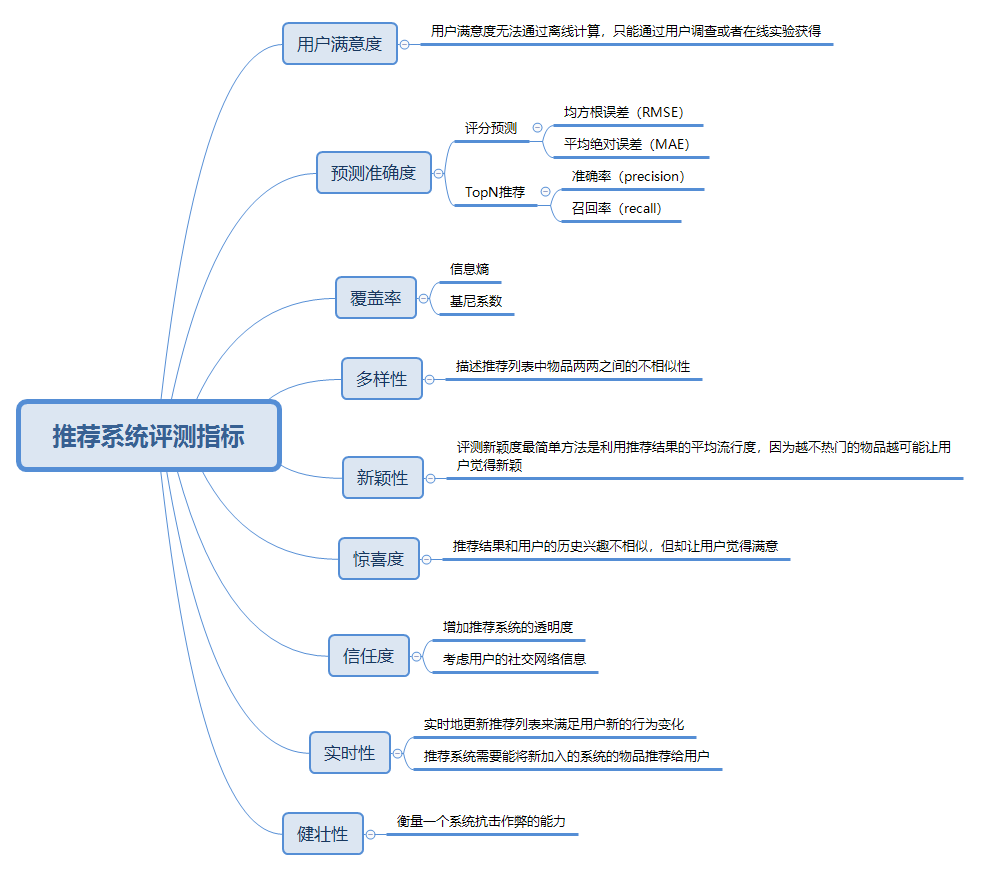1. 用户满意度（在线）

2. 预测准确度（离线）

2.1 评分预测

RMSE的定义为：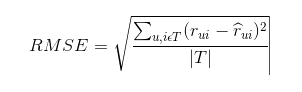MAE采用绝对值计算预测误差，它的定义为：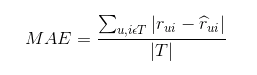2.2 TopN推荐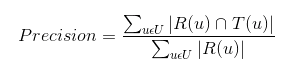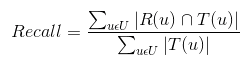3. 覆盖率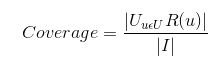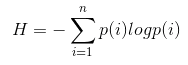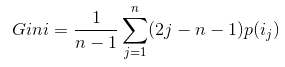4. 多样性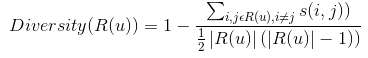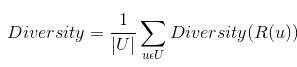5. 新颖性

6.惊喜度

7.信任度

8.实时性

9. 健壮性

1. 设计推荐系统时尽量使用代价比较高的用户行为。如在用户的购买行为和浏览行为中，显然购买行为的代价更高。

2. 在使用数据时，进行攻击检测，从而对数据进行清理。

SofaSofa数据科学社区DS面试题库 DS面经checclche   2018-11-27 12:38

3

SofaSofa数据科学社区DS面试题库 DS面经Arsjun   2018-02-19 14:59相关主题